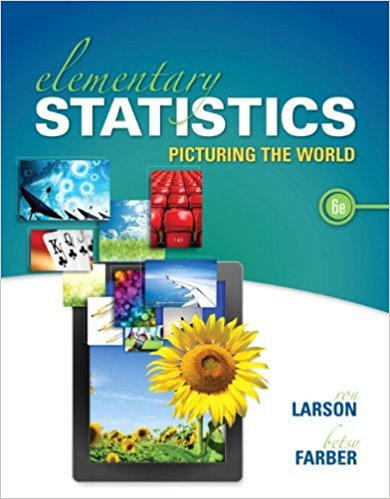×
×

# Solutions for Chapter 5.1: Introduction to Normal Distributions and the Standard Normal Distribution## Full solutions for Elementary Statistics: Picturing the World | 6th Edition

ISBN: 9780321911216Solutions for Chapter 5.1: Introduction to Normal Distributions and the Standard Normal Distribution

Solutions for Chapter 5.1
4 5 0 292 Reviews
24
5
##### ISBN: 9780321911216

Elementary Statistics: Picturing the World was written by and is associated to the ISBN: 9780321911216. This textbook survival guide was created for the textbook: Elementary Statistics: Picturing the World , edition: 6. Chapter 5.1: Introduction to Normal Distributions and the Standard Normal Distribution includes 60 full step-by-step solutions. This expansive textbook survival guide covers the following chapters and their solutions. Since 60 problems in chapter 5.1: Introduction to Normal Distributions and the Standard Normal Distribution have been answered, more than 107745 students have viewed full step-by-step solutions from this chapter.

Key Statistics Terms and definitions covered in this textbook

A variation of the R 2 statistic that compensates for the number of parameters in a regression model. Essentially, the adjustment is a penalty for increasing the number of parameters in the model. Alias. In a fractional factorial experiment when certain factor effects cannot be estimated uniquely, they are said to be aliased.

• Backward elimination

A method of variable selection in regression that begins with all of the candidate regressor variables in the model and eliminates the insigniicant regressors one at a time until only signiicant regressors remain

• Bimodal distribution.

A distribution with two modes

• Categorical data

Data consisting of counts or observations that can be classiied into categories. The categories may be descriptive.

• Central limit theorem

The simplest form of the central limit theorem states that the sum of n independently distributed random variables will tend to be normally distributed as n becomes large. It is a necessary and suficient condition that none of the variances of the individual random variables are large in comparison to their sum. There are more general forms of the central theorem that allow ininite variances and correlated random variables, and there is a multivariate version of the theorem.

• Components of variance

The individual components of the total variance that are attributable to speciic sources. This usually refers to the individual variance components arising from a random or mixed model analysis of variance.

• Confounding

When a factorial experiment is run in blocks and the blocks are too small to contain a complete replicate of the experiment, one can run a fraction of the replicate in each block, but this results in losing information on some effects. These effects are linked with or confounded with the blocks. In general, when two factors are varied such that their individual effects cannot be determined separately, their effects are said to be confounded.

• Consistent estimator

An estimator that converges in probability to the true value of the estimated parameter as the sample size increases.

• Continuous uniform random variable

A continuous random variable with range of a inite interval and a constant probability density function.

• Contrast

A linear function of treatment means with coeficients that total zero. A contrast is a summary of treatment means that is of interest in an experiment.

• Correction factor

A term used for the quantity ( / )( ) 1 1 2 n xi i n ? = that is subtracted from xi i n 2 ? =1 to give the corrected sum of squares deined as (/ ) ( ) 1 1 2 n xx i x i n ? = i ? . The correction factor can also be written as nx 2 .

• Degrees of freedom.

The number of independent comparisons that can be made among the elements of a sample. The term is analogous to the number of degrees of freedom for an object in a dynamic system, which is the number of independent coordinates required to determine the motion of the object.

• Dispersion

The amount of variability exhibited by data

• Enumerative study

A study in which a sample from a population is used to make inference to the population. See Analytic study

• Error of estimation

The difference between an estimated value and the true value.

• Error variance

The variance of an error term or component in a model.

• Experiment

A series of tests in which changes are made to the system under study

• F distribution.

The distribution of the random variable deined as the ratio of two independent chi-square random variables, each divided by its number of degrees of freedom.

• Factorial experiment

A type of experimental design in which every level of one factor is tested in combination with every level of another factor. In general, in a factorial experiment, all possible combinations of factor levels are tested.

• False alarm

A signal from a control chart when no assignable causes are present

×